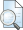## PENGARUH KINERJA KEUANGAN EMITEN TERHADAP FLUKTUASI HARGA SAHAM PERUSAHAAN MANUFAKTUR YANG LISTING DI BURSA EFEK INDONESIA

NUGRAHENI, NIKE DEWI (2008) PENGARUH KINERJA KEUANGAN EMITEN TERHADAP FLUKTUASI HARGA SAHAM PERUSAHAAN MANUFAKTUR YANG LISTING DI BURSA EFEK INDONESIA. Other thesis, University of Muhammadiyah Malang.Preview
Text

## Abstract

This research is explanatory study at company of manufactur which is listing in BEI with title “Influence of Performance Company’s Finance to Fluctuation Price Share Company of Manufacture Which Is Listing in BEI”. Intention of this research is to know how big influence of variable current ratio, debt to equity ratio, return on equity and total assets turn over; and to know variable which having strongest influence to share price at company of manufacture which was listing in BEI. In this research, writer take hypothesis, that is; variable current ratio, debt to equity ratio, return on equity, total asset turn over have influence which isn’t if to fluctuation sharp price and current ratio which having strongest influence to Company of manufacture. Analyzer which used in this research is analysis of regression doubled linear, pursuant to result test F known that result analyze obtained on value of Fhitung = 8,652 and storey : level of signification F = 0,00. value of Fhitung = 8,652 bigger compared to with value of Ftabel (4,46) = 2,53 (Fhitung > Ftabel) and storey; level of signification F(0,00) is smaller the than α = 0,05 (sign F), so can be concluded that variable of current ratio, debt to equity ratio, return on equity, total asset turn over by together or by simultan have influence is it to share price company of manufacture. In this case statistically hypothesis zero (H0) refused and accept alternative hypothesis (Ha) Pursuant to statistical test result (test of t), known that variable of x2 (debt to equity ratio) with value of sign t = 0,000 α = 0,05 hence (tsign = α) = (0,000 < 0,05) or (t hitung > t tabel) = (5,197 > 2,000). The mentioned indicate that by partial variable of debt to equity ratio have an effect on isn’t it to fluctuation share price. While at variable of x1 (current ratio), x3 (return on equity), x4 (total asset turn over) by partial do not have influence, which isn’t it to variable of Y (fluctuation share price). Beta value (coefficient of regression) variable of current ratio = 0,052; debt to equity ratio = 0,549; return on equity = 0,166; total asset turn over = 0,098 from four variable, variable of debt to equity ratio biggest beta value, so that can be said that by variable of x2 have most dominant influence to y variable (fluctuation price share). Pursuant to result analyze above, writer suggest that company in improving share price can pay attention variable having most dominant influence to share price in this research

Item Type: Thesis (Other) H Social Sciences > HB Economic Theory Faculty of Economics and Business > Department of Accounting (62201) Anggit Aldila 21 May 2012 06:28 21 May 2012 06:28 http://eprints.umm.ac.id/id/eprint/5616

### Actions (login required)View Item